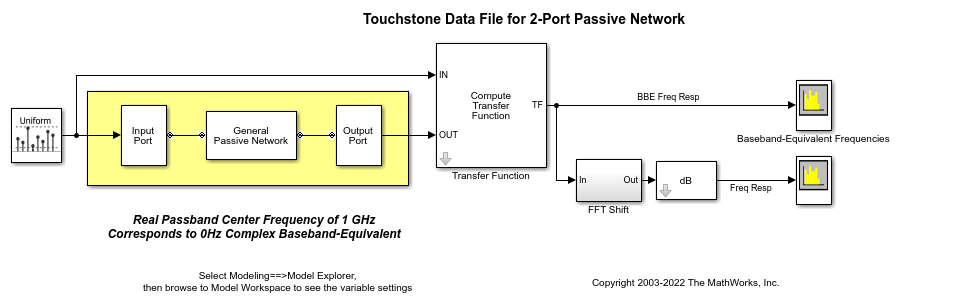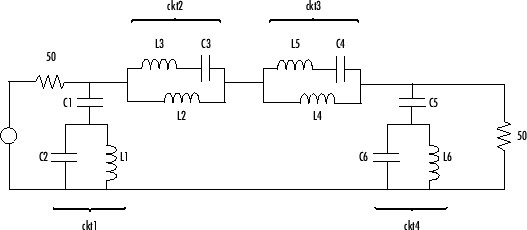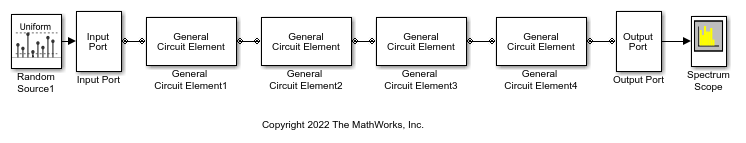# Specify or Import Component Data

This example shows how to specify or import component data in and equivalent baseband system.

### Specify Parameter Values

You can set block parameter values either

### Supported File Types for Importing Data

RF blockset™ lets you import the following types of data files:

• Industry-standard file formats — Touchstone S2P, Y2P, Z2P, and H2P formats specify the network parameters and noise information for measured and simulated data. For more information, see Touchstone specifications.

• Agilent® P2D file format — Specifies amplifier and mixer large-signal, power-dependent network parameters, noise data, and intermodulation tables for several operating conditions, such as temperature and bias values. The P2D file format lets you import system-level verification models of amplifiers and mixers.

• Agilent S2D file format — Specifies amplifier and mixer network parameters with gain compression, power-dependent S21 parameters, noise data, and intermodulation tables for several operating conditions. The S2D file format lets you import system-level verification models of amplifiers and mixers.

• MathWorks™ amplifier (AMP) file format — Specifies amplifier network parameters, power data, noise data, and third-order intercept point. For more information, see AMP File Data Sections.

• MATLAB® circuits — RF Toolbox™ circuit objects in the MATLAB workspace specify network parameters, noise data, and third-order intercept point information of circuits with different topologies. For more information, see RF Circuit Objects.

### Import Data Files into RF Blocks

RF blockset lets you import industry-standard data files, Agilent P2D and S2D files, and MathWorks AMP files into specific blocks to simulate the behavior of measured components in the Simulink modeling environment.

This section contains the following topics:

• Blocks Used to Import Data

• How to Import Data Files

### Blocks Used to Import Data

Three blocks in the Physical library accept data from a file. The following table lists the blocks and any corresponding data format that each supports.### How to Import Data Files

To import a data file:

• Choose the block that best represents your component from the list of blocks that accept file data shown in Blocks Used to Import Data section.

• Open the Physical library, and navigate to the sublibrary that contains the block.

• Click and drag the block into your Simulink model.

• In the block dialog box, enter the name of your data file for the Data file parameter. The file name must include the extension. If the file is not in your MATLAB path, specify the full path to the file or use the Browse button to find the file.

### Example: Import Touchstone Data File into RF Model

In this example, you model the frequency response of a passive component using data from a Touchstone file, `defaultbandpass.s2p`.

You use a model from one of the RF Blockset Equivalent Baseband examples to perform the following tasks:

• Import Data into General Passive Network Block

• Validate Passive Component

### Import Data into General Passive Network Block

In this part of the example, you inspect the `defaultbandpass.s2p` file and import data into the RF model using the General Passive Network block.

Type the following at the MATLAB prompt to open the `defaultbandpass.s2p` file:

`edit defaultbandpass.s2p`

Open `sparam_filter.slx`, Touchstone Data File for 2-Port Bandpass Filter.

```open_system("sparam_filter.slx") ```Double-click the General Passive Network block to display its parameters.

The Data source parameter is set to `Data file`, to specify the data file to import. The Data file parameter is set to `defaultbandpass.s2p`. The block uses this data with the other block parameters during simulation.

Note that When the imported file contains data that is measured at frequencies other than the modeling frequencies, use the Interpolation method parameter to specify how the block determines the data values at the modeling frequencies. For more information, see Determine Modeling Frequencies and Map Network Parameters to Modeling Frequencies.

### Validate Passive Component

In this part of the example, you plot the network parameters of the General Passive Network block to validate the data you imported in Import Data into General Passive Network Block.

• Open the General Passive Network block dialog box, and select the Visualization tab.

• Set the Source of frequency data parameter to `User-specified`.

• Set the Frequency data (Hz) parameter to `[0.5e9:0.1e6:1.5e9]`.

• Click Plot.

These actions create a plot of the magnitude and phase of S21 as a function of frequency.### Import Circuits from MATLAB Workspace

You can only connect Physical library blocks in cascade. However, the blockset works with RF Toolbox software to let you include additional circuit topologies in an RF model. To model circuit topologies that contain other types of connections, you must define a circuit in the MATLAB workspace and import it into an RF model.

To import a circuit from the MATLAB workspace:

• Define the circuit object in the MATLAB workspace using the RF Toolbox functions. For more information, see RF Circuit Objects.

• Add a General Circuit Element block to your RF model from the Black Box Elements sublibrary of the Physical library. For information on how to open this library, see Equivalent Baseband Library.

• Enter the circuit object name in the RFCKT object parameter in the General Circuit Element block dialog box.

This procedure is illustrated by example in the following section.

### Example: Import Bandstop Filter into RF Model

In this example, you simulate the frequency response of a filter that you model using circuit objects from the MATLAB workspace.

The filter in this example is the 50-ohm bandstop filter shown in the following figure.You represent the filter using four circuit objects that correspond to the four parts of the filter, `ckt1`, `ckt2`, `ckt3`, and `ckt4` in the diagram. You use an input signal with random, complex input values that have a Gaussian distribution to stimulate the filter. The scope block displays the output signal.

This example illustrates how to perform the following tasks:

• Create Circuit Objects in MATLAB Workspace

• Build Model

• Specify and Import Component Data

• Run Simulation and Plot Results

### Create Circuit Objects in MATLAB Workspace

In this part of the example, you define MATLAB variables to represent the physical properties of the filter shown in the previous figure, Bandstop Filter Diagram, and use functions from RF Toolbox software to create RF circuit objects that model the filter components.

Type the following at the MATLAB prompt to define the filter's capacitance and inductance values in the MATLAB workspace:

```C1 = 1.734e-12; C2 = 4.394e-12; C3 = 7.079e-12; C4 = 7.532e-12; C5 = 1.734e-12; C6 = 4.394e-12; L1 = 25.70e-9; L2 = 3.760e-9; L3 = 17.97e-9; L4 = 3.775e-9; L5 = 17.63e-9; L6 = 25.70e-9; ```

Type the following at the MATLAB prompt to create RF circuit objects that model the components labeled `ckt1`, `ckt2`, `ckt3`, and `ckt4` in the circuit diagram:

```ckt1 = ... rfckt.series('Ckts',{rfckt.shuntrlc('C',C1),... rfckt.shuntrlc('L',L1,'C',C2)}); ckt2 = ... rfckt.parallel('Ckts',{rfckt.seriesrlc('L',L2),... rfckt.seriesrlc('L',L3,'C',C3)}); ckt3 = ... rfckt.parallel('Ckts',{rfckt.seriesrlc('L',L4),... rfckt.seriesrlc('L',L5,'C',C4)}); ckt4 = ... rfckt.series('Ckts',{rfckt.shuntrlc('C',C5),... rfckt.shuntrlc('L',L6,'C',C6)}); ```

For more information about the RF Toolbox objects used in this example, see the `rfckt.series`, `rfckt.parallel`, `rfckt.seriesrlc`, and `rfckt.shuntrlc` object reference pages in the RF Toolbox documentation.

### Build Model

In this portion of the example, you create a Simulink model. For more information about adding and connecting components, see Model RF Components.

Create a new model. Add to the model the blocks shown in the following table. The Library column of the table specifies the hierarchical path to each block.Connect the blocks as shown in the following figure. Change the names of your General Circuit Element blocks to match those in the figure by double-clicking the text below the block and typing a new name. Alternatively, open the model attached in this example.

```open_system('importing_circuits.slx') ```### Specify and Import Component Data

In this portion of the example, you specify block parameters. To open the parameter dialog box for each block, double-click the block.

In the Random Source block, set:

• Source type`Gaussian`

• Sample time`1/100e6`

• Samples per frame`256`

• Complexity`Complex`

Selecting these settings creates an input signal with random, complex input values that have a Gaussian distribution.

In the Input Port block, set:

• Treat input Simulink signal as`Source voltage`

• Finite impulse response filter length`256`

• Center frequency (Hz)`400e6`

• Sample time`1/100e6`

• Input processing — Columns as channels (frame based)

and clear the Add noise check box. Selecting these settings defines the physical characteristics and modeling bandwidth of the filter.

Set the parameters of the General Circuit Element blocks as follows:

• In the General Circuit Element1 block, set the RFCKT object parameter to `ckt1`

• In the General Circuit Element2 block, set the RFCKT object parameter to `ckt2`

• In the General Circuit Element3 block, set the RFCKT object parameter to `ckt3`

• In the General Circuit Element4 block, set the RFCKT object parameter to `ckt4`

Selecting these settings imports the circuit objects that model the filter components into the model.

In the Output Port block, set the Load impedance (ohms) parameter to `50`.

Set the Spectrum Analyzer block parameters as follows:

• In the View tab, under `Spectrum Settings` > `Main options`, set Type to Power denisity, Method to `Welch` , and Window Length to `1024` and NFFT to `256`

• In the View tab, under `Spectrum Settings` > `Trace options`, set the Averaging method to `Running` and Averages to `100`. This parameter establishes the number of spectra that the scope averages to produce the displayed signal. You use a value of 100 because the input signal is random and you want to display the average filter response over a large number of input values.

• In the View tab, under `Configuration Properties`, set the Y-limit (Minimum) parameter to `-115` and the Y-limit parameter (Maximum) to `-76.4`. These values set the range of x- and y-values on the display such that the entire signal is visible when you run the simulation.

• Set the Y label parameter to `Magnitude-squared`.

### Run Simulation and Plot the Results

Click Run in the model window to start the simulation.

```sim("importing_circuits.slx") ```The Spectrum Scope block displays the frequency response at the shifted (baseband-equivalent) frequencies, not at the selected passband frequencies. You can relabel the x-axis of the Spectrum Scope window to display the passband signal by entering the Center frequency parameter value of `400e6` (from the Input Port block) for the Frequency display offset (Hz) parameter in the Axis Properties tab of the Spectrum Scope block. For more information on complex-baseband modeling, see Create Complex Baseband-Equivalent Model.

 Geffe, P.R., “Novel designs for elliptic bandstop filters,” RF Design, February 1999.